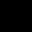# If on an item a company gives 25% discount, they earn 25% profit. If they now give 10% discount then what is the profit percentage.(a) 40%(b) 55%(c) 35%(d) 30%

## One Answer on this Question

1.Soumyadip Dutta says:

let MP = 100x
25% discount = 75x -> SP
Profit% = (Profit/CP)x100
125 = (75x/CP)x100
.: CP = 60x

Now if discount = 10%
SP = 90x
.: Profit% = ((90x-60x)/60x)x100 = 50%

Scroll to top